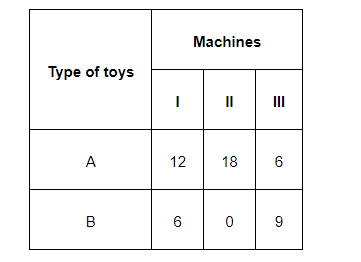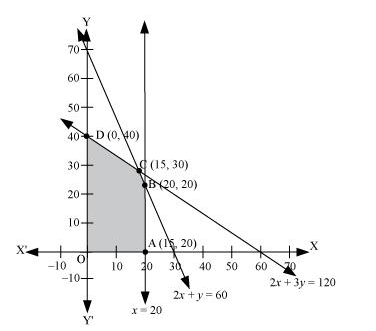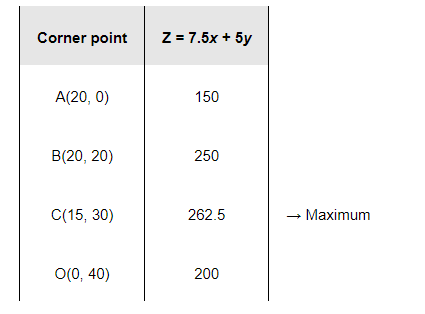# A manufacturer makes two types of toys A and B.

Question:

A manufacturer makes two types of toys A and B. Three machines are needed for this purpose and the time (in minutes) required for each toy on the machines is given below:Each machine is available for a maximum of 6 hours per day. If the profit on each toy of type A is Rs 7.50 and that on each toy of type B is Rs 5, show that 15 toys of type A and 30 of type B should be manufactured in a day to get maximum profit.

Solution:

Let x and y toys of type A and type B respectively be manufactured in a day.

The given problem can be formulated as follows.

Maximize $z=7.5 x+5 y$                      (1)

subject to the constraints,

$2 x+y \leq 60$                                    (2)

$x \leq 20$ (3)

$2 x+3 y \leq 120$                               (4)

$x, y \geq 0$                                        (5)

The feasible region determined by the constraints is as follows.The corner points of the feasible region are A (20, 0), B (20, 20), C (15, 30), and D (0, 40).

The values of z at these corner points are as follows.The maximum value of z is 262.5 at (15, 30).

Thus, the manufacturer should manufacture 15 toys of type A and 30 toys of type B to maximize the profit.